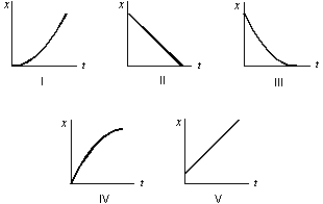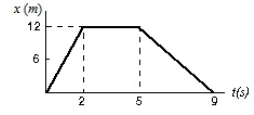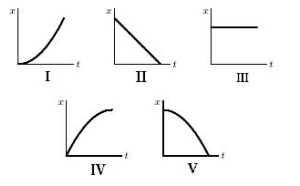Motion Along a Straight Line

# Fundamentals of Physics Study Set 2

Physics & Astronomy

## Quiz 2 :Motion Along a Straight LineStudy FlashcardsLooking for Introductory Physics Homework Help?## Quiz 2 :Motion Along a Straight Line

Showing 1 - 20 of 90When can you treat a moving object as if it were a point-like particle?
Free
Multiple Choice

DA particle moves along the x axis from xi to x f .Of the following values of the initial and final coordinates, which results in the displacement with the largest magnitude?
Free
Multiple Choice

EA particle moves along the x axis from xi to x f .Of the following values of the initial and final coordinates, which results in a negative displacement?
Free
Multiple Choice

BA particle moves from point x1 to point x2.Its displacement is given by:
Multiple ChoiceA car starts from Hither, goes 50 km in a straight line to Yon, immediately turns around, and returns to Hither.The time for this round trip is 2 hours.The magnitude of the average velocity of the car for this round trip is:
Multiple ChoiceThe coordinate of an object is given as a function of time by x = 7t - 3t2, where x is in meters and t is in seconds.Its average velocity over the interval from t = 0 to t = 2 s is:
Multiple ChoiceThe position y of a particle moving along the y axis depends on the time t according to the equation y = at - bt2.The dimensions of the quantities a and b are respectively:
Multiple ChoiceThe average speed of a moving object during a given interval of time is always:
Multiple ChoiceTwo automobiles are 150 kilometers apart and traveling toward each other.One automobile is moving at 60 km/h and the other is moving at 40 km/h.In how many hours will they meet?
Multiple ChoiceA car travels 40 kilometers at an average speed of 80 km/h and then travels 40 kilometers at an average speed of 40 km/h.The average speed of the car for this 80 km trip is:
Multiple ChoiceA car starts from Hither, goes 50 km in a straight line to Yon, immediately turns around, and returns to Hither.The time for this round trip is 2 hours.The average speed of the car for this round trip is:
Multiple ChoiceYou leave your house and drive to your friend's house, where you stay a while.Then you come back home.Which of the following must be true of your trip?
Multiple ChoiceWhich of the following five coordinate versus time graphs represents the motion of an object whose speed is increasing?Multiple ChoiceThis graph shows the position of a particle as a function of time.What is its average velocity between t = 5s and t = 9s?Multiple ChoiceThe coordinate of a particle in meters is given by x(t)= 16t - 3.0t3, where the time t is in seconds.The particle is momentarily at rest at t =
Multiple ChoiceEach of four particles moves along an x axis.Their coordinates (in meters)as functions of time (in seconds)are given byFor which of these particles is the velocity increasing for t > 0?
Multiple ChoiceThe coordinate of an object is given as a function of time by x = 7t - 3t2, where x is in meters and t is in seconds.Its velocity at t = 3s is:
Multiple ChoiceWhich of the following five coordinate versus time graphs represents the motion of an object moving with a constant nonzero speed?Multiple ChoiceThis graph shows the position of a particle as a function of time.What is its instantaneous velocity at t = 7s?